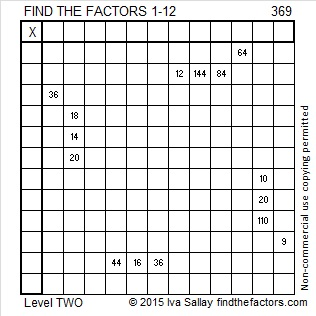# 369 and Level 2

3, 6, & 9 are each divisible by 3, so 369 is composite and a multiple of 3. Scroll down past the puzzle to see its factors.Print the puzzles or type the factors on this excel file:12 Factors 2015-01-26

• 369 is a composite number.
• Prime factorization: 369 = 3 x 3 x 41, which can be written 369 = (3^2) x 41
• The exponents in the prime factorization are 2 and 1. Adding one to each and multiplying we get (2 + 1)(1 + 1) = 3 x 2  = 6. Therefore 369 has exactly 6 factors.
• Factors of 369: 1, 3, 9, 41, 123, 369
• Factor pairs: 369 = 1 x 369, 3 x 123, or 9 x 41
• Taking the factor pair with the largest square number factor, we get √369 = (√9)(√41) = 3√41 ≈ 19.209